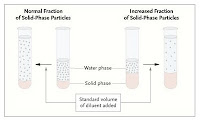# Sodium – interpretation of measurements Part IIOk, so here is the brief review of the various clinical methods of detecting sodium concentration (as I understand it):

Flame photometer
The older flame photometer method measured the concentration of sodium in plasma. The preparation of the sample involved dilution of the plasma, and assumed that plasma water was 93% of total plasma.

Imagine that the lab reports a sodium concentration of 143mmol/L. Assume that the plasma water is 93% of total plasma – then the sodium concentration in plasma water would be 143/0.93 = 154mmol/L. Let’s take this as ‘normal plasma water sodium’.

Now imagine that the sodium measurement in another patient came back from the lab at 120mmol/L:
– if plasma water is 93% of total plasma, then the sodium in plasma water is 120/0.93 = 129mmol/L – true hyponatraemia
– if plasma water is only 80% of total plasma, then the sodium in plasma water is 120/0.8 = 150mmol/L. This is closer to what we’d expect in normal circumstances, and so we can call this pseudohyponatraemia.

Indirect Ion Selective Electrode
Ion-selective electrodes (ISEs) only measure free sodium ions in a solution; not the volume in which they are dissolved.

The indirect method involves a dilution of the acquired total plasma sample– and again, the lab assumes that plasma water is 93% of total plasma. Therefore the potential for pseudohyponatraemia unfortunately still exists if your lab performs these types of measurements.

Direct Ion Selective Electrode
The direct method of ISE measurement does not use a dilution step and has no assumptions regarding the percentage plasma water of total plasma. Direct measurements are most commonly used in blood gas analyzers. Remember, that direct ISEs measure activity.

Entropy suggests that as energy is introduced to a system, it will be dispersed in a uniform pattern to allow a state of maximal disorder (the lowest energy state). Introduction of sodium (solute) to a solution (blood) does not follow this classic law, as the sodium can interact with water molecules and other ions. Therefore, not all sodium ions are free to enter a reaction, which explains why the concentration of sodium is different than the measured activity. Biochemists multiply the absolute concentration by an activity coefficient (around 0.93 for sodium) to determine the actual activity of sodium.

The direct ISE will only measure free sodium ions, which are limited to the plasma water fraction. From our assumptions above, we noted that a ‘normal person’ had a plasma water sodium concentration of 154mmol/L. But, because not all these sodium ions are free to react, then the measured activity of sodium in plasma water will be around 143mEq/L. This corresponds almost perfectly with the figure obtained by multiplying the sodium concentration in plasma water by the activity coefficient of sodium (154 x 0.93 = 143mEq/L). Note that this 0.93 comes from the activity coefficient of sodium, not the plasma water fraction – it just so happens that they are similar.
Because the direct ISE does not make any assumptions about the plasma water content, it is immune from the concept of pseudohyponatraemia.

Finally, back to our patient with the lab reported sodium of 120mmol/L, who has 93% plasma water, and from our earlier calculations had a plasma water sodium of 129mmol/L. If we measure the sodium activity using direct ISE, we would find that the sodium activity equals the plasma water sodium multiplied by the activity coefficient (129 x 0.93) = 120mmol/L – again true hyponatraemia.

### 1 comment

1.I would like help with the following.

1. Assume that a plasma sample has a [Na] of 140 mEq/L plasma as measured by flame photometry and 150 mEq/L plasma water when corrected for solids content.

What would be the direct ISE reading on modern blood-gas machines?

2. Assume that a carefully prepared NaCl solution has a [Na] of 150 mEq/L H2O. What would be the [Na] measured on the same machine?